# Simpson formula

(diff) ← Older revision | Latest revision (diff) | Newer revision → (diff)

A special case of the Newton–Cotes quadrature formula, in which three nodes are specified: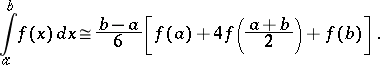(1)

Let the intervalbe broken up into an even numberof subintervals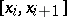,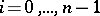, of length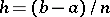, and calculate the integral over the intervalby the quadrature formula (1):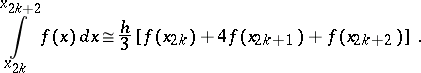Summation overfrom 0 to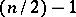on both sides leads to the composite Simpson formula(2)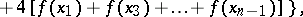where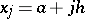,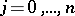. The quadrature formula (2) is also called Simpson's formula (that is, the word composite is dropped). The algebraic degree of accuracy of (2), and of (1), is equal to 3.

If the integrandhas a continuous derivative of the fourth order on, then the error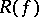of the quadrature formula (2) — the difference between the left-hand and right-hand members of the approximate equation (2) — can be written as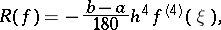whereis some point in the interval.

Simpson's formula was named after Th. Simpson, who obtained it in 1743, although the formula was already known, for example to J. Gregory, in 1668.